Home » SQL SELECT INTO SELECT

# SQL SELECT INTO SELECTSQL SELECT INTO statement copies data from one table into new table.

The new table will be created with the same column names and data types as defined in the old table .

SYNTAX

```SELECT Column_name1, Column_name2, ..
INTO new_table
FROM old_table
WHERE [Condition]  ```

Lets, look at an example of  SELECT INTO SELECT in SQL .

Here, we have a sample table named as DEPT in database Prod_Db as given below.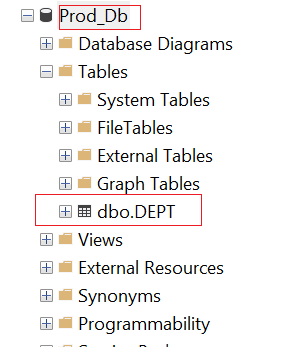Following are the Create table and Insert records into table scripts.

`CREATE TABLE DEPT (DEPT_ID INT NOT NULL, DEPT_NAME VARCHAR(100), DEPT_CODE VARCHAR(30))INSERT INTO DEPT(DEPT_ID, DEPT_NAME, DEPT_CODE)VALUES( 501, 'IT', 001),(502, 'FINANCE', 002),(503, 'HR', 003),(504, 'MIS', 004),(505, 'CALL CENTER', 005),(506, 'SALES', 006)`

Suppose, you want to insert all the records present in table DEPT into new table named as DEPT_LOG.

Before that just take a look on table DEPT and DEPT_LOG.

`SELECT * FROM DEPT`While table DEPT_LOG does not exists in database.

`SELECT * FROM DEPT_LOG`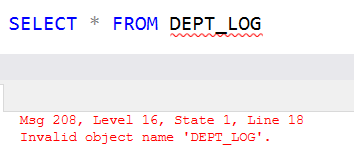As you have seen above , there are six records in DEPT table while DEPT_LOG table does not even exist in database.

Now, our requirement is that to copy all the data present in DEPT table into DEPT_LOG table

Following SQL statement, will create a new table named as DEPT_LOG with same column name and schema as defined in DEPT table and then copy all the data present in DEPT table into table DEPT_LOG table.

`SELECT DEPT_ID, DEPT_NAME, DEPT_CODE INTO DEPT_LOG FROM DEPT;`

You can see, commands completed successfully and 6 rows are affected.Lets, verify the records in DEPT_LOG table with DEPT table.

`SELECT * FROM DEPTSELECT * FROM DEPT_LOG`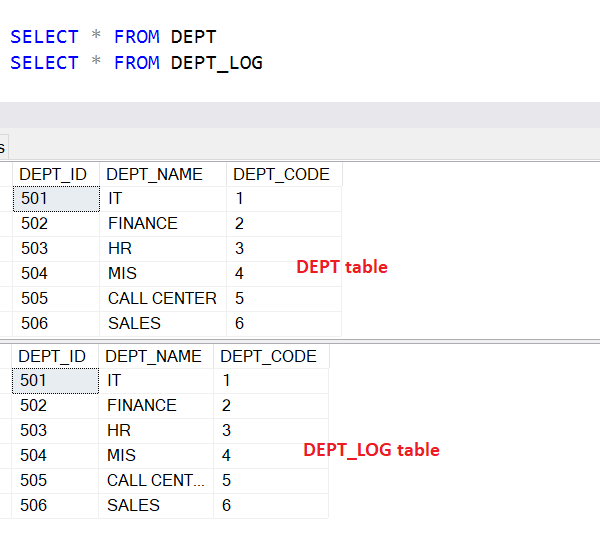As you can see DEPT_LOG table is now exists in database and contains same number of records as DEPT table.

You can also verify whether a table DEPT_Log is created or not in database using object explorer.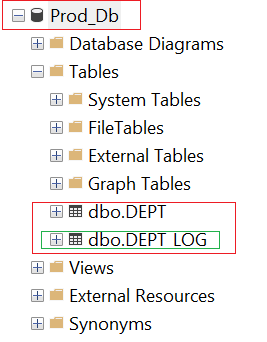If you want to copy only specific records then you can also use WHERE clause in SELECT INTO Statement.

Lets say you want to copy only a data for IT department into new table then you can use WHERE clause to filter the records in table as given below.

`SELECT DEPT_ID, DEPT_NAME, DEPT_CODE INTO DEPT_LOG FROM DEPT WHERE DEPT_Name = 'IT'`

#### Copy only table schema using SELECT INTO

In case if you just want to copy the table schema but do not want to copy the data, It can also be done using SELECT INTO statement by specifying the any condition that returns false such as providing a condition 1=0 in WHERE clause.

As 1 can not be equal to 0, and that is false so for this condition no data is returned from a table, which means only a new table is created in database with out copying of the data of table into new table.

Here, you can use any condition in WHERE clause but make sure that for given condition query should not return any data other wise new table will be created with data.

Lets create a new table named as DEPT_LOG1 that will have a same schema as DEPT table.

You can see DEPT_LOG1 table does not exist in Database in object explorer.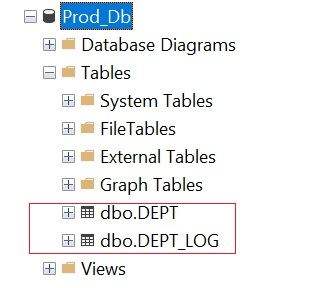Lets verify the same using T-SQL statement.

`SELECT * FROM DEPT_LOG1`Lets create a table DEPT_LOG1 in database that will have a same schema of DEPT table. But this table will not have any data.

`SELECT DEPT_ID, DEPT_NAME, DEPT_CODE INTO DEPT_LOG1FROM DEPTWHERE 1=0;`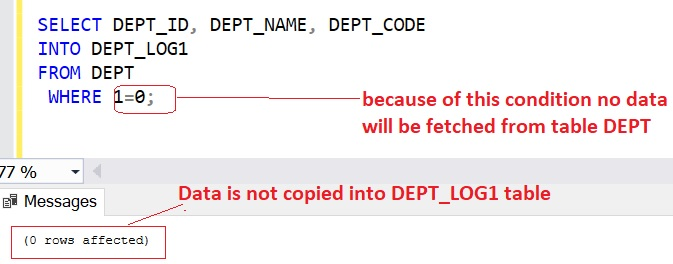Lets verify the DEPT_LOG1 table structure, and you can see table contains same numeber of columns as DEPT table.Lets select the records from DEPT_Log1 table.

`SELECT * FROM DEPTSELECT * FROM DEPT_LOG1`Now you can see DEPT_LOG1 is created in database without copying the data from DEPT table, only a table schema is copied.This site uses Akismet to reduce spam. Learn how your comment data is processed.